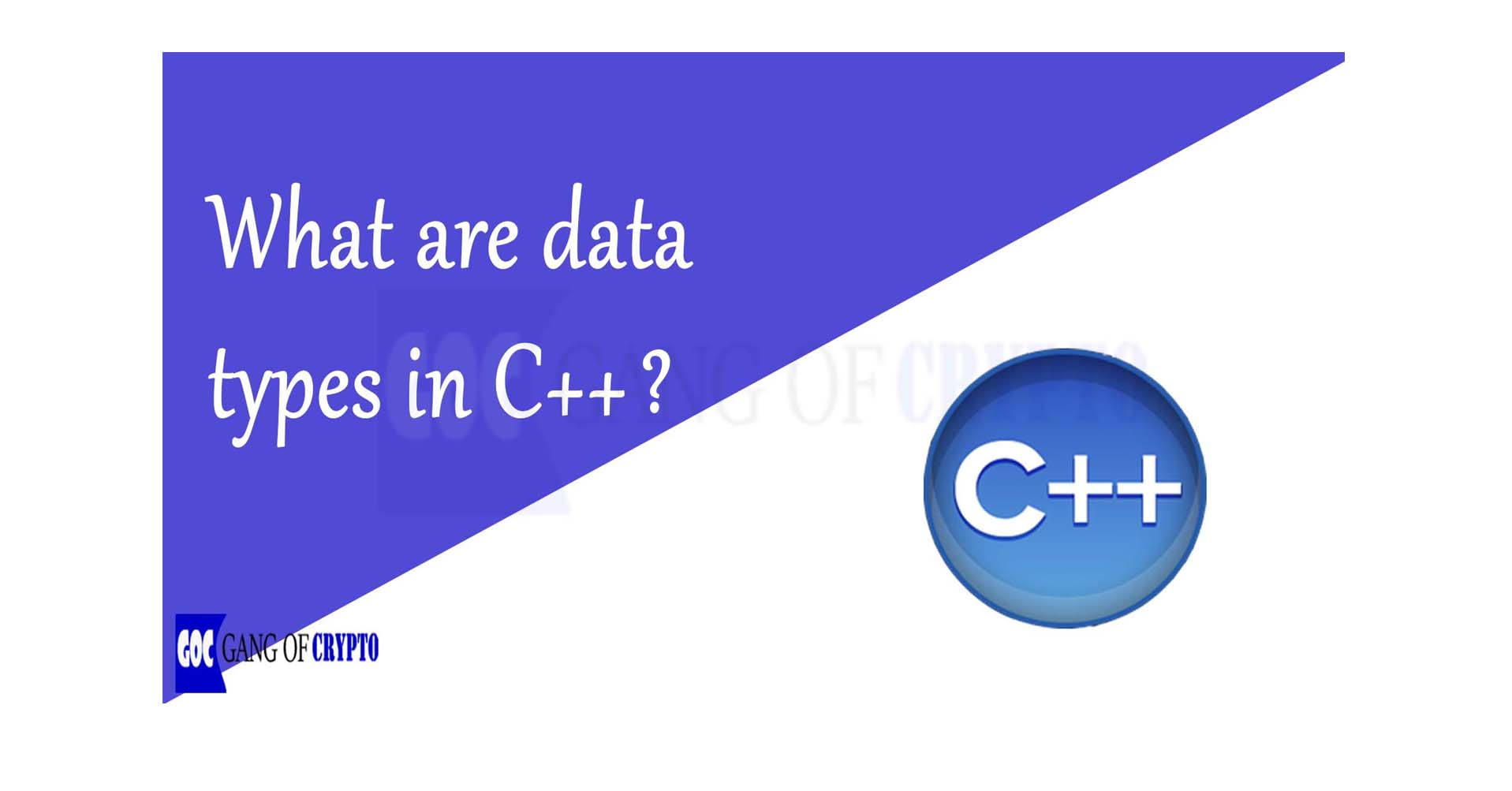# Data Types in C++

## Introduction to C++ Data Types

A data type is used because to let know the variable, what type of element it is, and going to determine the memory allocation. There are three different C++ data types namely: Primitive, Derived, and User Defined.

## Data Types in C++

There are three different data types in C++ which are explained below:

### a) Primitive Data Types

These data types are pre-defined in C++ and these are also called built-in data types. We can directly use these data types to declare the variables.

1. Integer:  These are defined by “int”. The size of memory allocated for the “int” data type can be 2 or 4 bytes.
2. Character:  These are defined by “char”. The size of memory allocated for the “char” data type can be known 1 byte.
3. Floating Point: These are defined by “float”. The size of memory allocated for the “float” data type can be 4 or 8 bytes.
4. String:  These are defined by “string”. The size of memory allocated for the “string” . The size of String can be 16 or 32.

Size of every Data Type is known dynamically by operator Sizeof(). [ here. ]

#### Program 1: Program using primitive Data types in C++

``````#include<iostream>
using namespace std;
int main(){
//Initializing Data types
int i;
char ch;
float f;
string s;
//Giving Values to Variables
i = 10;
ch = 'A';
f = 1.245;
s = "The_coder";
//Displaying values of different Data types
cout<<"\n Integer is "<<i;
cout<<"\n Character is "<<ch;
cout<<"\n Float is "<<f;
cout<<"\n String is"<<s;

} ``````
OUTPUT:
Integer is 10
Character is A
Float is 1.245
String is The_Coder

### b) Derived Data Types

These data types are derived from the primitive data types, which justifies its name.

1. Array: An array is a collection of data items that are of the same type and accessed using a common name.
2.  Pointer:  A pointer is a variable that stores the address of another variable.

#### Program 2: Program using Derived Data types in C++

``````#include<iostream>
using namespace std;
int main(){

//Initializing Array
int Arr = {1,2,3,4,5};

//Initializing Pointer
int a=10;
int *ptr;
ptr = &a;

//Displaying array without LOOP
cout<<"\n Array element 1 is" << Arr;
cout<<"\n Array element 2 is" << Arr;
cout<<"\n Array element 3 is" << Arr;
cout<<"\n Array element 4 is" << Arr;
cout<<"\n Array element 5 is" << Arr;

//Displaying Pointer
cout<<"\n Value  of a is "<<a;
cout<<"\n Value of pointer is "<<*ptr;
cout<<"\n Value of address of a stored in pointer is "<<ptr;

} ``````

OUTPUT:

Array element 1 is 1
Array element 2 is 2
Array element 3 is 3
Array element 4 is 4
Array element 5 is 5

Value of a is 10

Value of pointer is 10
Value of address of a stored in the pointer is 0x7ffc42667494

### c) User-Defined Data Types

The name itself says that these are the data types which the user can define. Let us see some examples here.

1. Structures: The structure is a collection of variables of different data types declared by a single name. Here is an example below.
2.  Class: A class is a user-defined data type, which holds its own data member functions, which can be accessed and used by creating an instance of that class.
3.  TypeDef: The typedef keyword allows the user to create a new name for data types such as int etc….
4.  Enumeration: It is defined by the word “enum”. These are generally used when we already know a set of values for a particular variable and choose a single value from them. Let’s have one example.

## Conclusion

I hope you enjoyed a lot while learning about data types of C++. We have covered many of them. Without declaring variables with specific data types, we cannot know the exact memory allocations and what set of instructions that a program has to do. You can try using different data types with modifiers and check how they work.